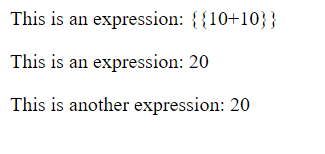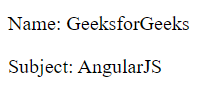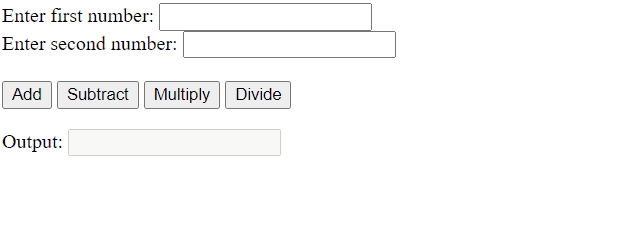# Explain ng-app Directive in AngularJS

• Difficulty Level : Expert
• Last Updated : 25 Feb, 2022

In this article, we will see the ng-app directive in AngularJS and will understand its use with the help of examples.

ng-app directive defines the beginning of an AngularJS application. It initializes the AngularJS framework and specifies the root element of the AngularJS application. Every AngularJS application must contain a root element. After an HTML document is loaded, the AngularJS framework searches for the ng-app directive in the page, if found it bootstraps itself and compiles the HTML template. Though you can specify the ng-app directive in any DOM element it is advised to specify the ng-app directive in the root of an HTML document.

Syntax:

`<element ng-app=""></element>`

Example 1: This example describes the use of the ng-app directive that helps to initialize the Angular JS application as the root element.

## HTML

 ```<``html``>` `<``head``>``    ``<``title``>ng-app directive``    ``<``script` `src``=``"https://cdnjs.cloudflare.com/ajax/libs/angular.js/1.4.7/angular.min.js"``>``    ````` `<``body``>``    ``<``div``>``        ` `<``p``>This is an expression: {{10+10}}` `    ````    ``<``div` `ng-app``=``""``>``        ` `<``p``>This is an expression: {{10+10}}` `        ``<``div``>``            ` `<``p``>This is another expression: {{10+10}}` `        ````    ```````

Explanation: In this example, there are 2 <div> tags. In the first <div> tag, there is a paragraph tag that has a message and an AngularJS expression. But in the output, we can observe that the expression is not evaluated. This is because the ng-app directive is not specified in this tag. In the other hand, the ng-app directive is specified in the second <div> tag, so the expressions in that tag are evaluated and the value is printed accordingly. So we can say that the AngularJS framework will only process the DOM elements and their child elements where the ng-app directive is applied.

Output:ng-app directive

Using ng-app with a module: We can also specify a module inside the ng-app directive. A module is a container for the different parts of an application. It can have controllers, directives, factories, services, etc.

Syntax:

`<element ng-app="myApp"></element>`

Example 2: In this example, we have specified the ng-app directive with a module named “myApp”

## HTML

 ```<``html``>` `<``head``>``    ``<``title``>Using ng-app with a module` `    ``<``script` `src``=``"https://cdnjs.cloudflare.com/ajax/libs/angular.js/1.4.7/angular.min.js"``>``    ````` `<``body``>``    ``<``div` `ng-app``=``"myApp"` `ng-controller``=``"myCtrl"``>``        ` `<``p``>Name: {{student.name}}` `        ` `<``p``>Subject: {{student.subject}}` `    ````    ``<``script` `type``=``"text/javascript"``>``        ``var app = angular.module("myApp", []);``        ``app.controller("myCtrl", function(\$scope) {``            ``\$scope.student = {``                ``'name': 'GeeksforGeeks',``                ``'subject': 'AngularJS'``            ``}``        ``});``    ```````

Explanation: In the above example, in the <div> tag, since we have specified a module named “myApp” in the ng-app directive, we are able to use the controller named “myCtrl” and its properties like the student object.

Output:ng-app directive with a module

Note: An AngularJS application, we can have only one ng-app directive, at a moment. If this directive is used more than once in the application then the first occurrence will be considered.

Example 3: In this example, the user can perform addition, subtraction, multiplication, and division of two numbers.

## HTML

 ```<``html``>` `<``head``>``    ``<``title``>``        ``Performing arithmetic operation with ng-app directive``    ````    ``<``script` `src``=``"https://ajax.googleapis.com/ajax/libs/angularjs/1.6.9/angular.min.js"``>``    ````` `<``body` `ng-app``=``"myApp"` `ng-controller``=``"myCtrl"``>``    ``<``form``>``        ``<``label``>Enter first number: ``        ``<``input` `type``=``"number"` `min``=``"0"` `ng-model``=``"number1"``>``        ``<``br``>``        ``<``label``>Enter second number:``        ``<``input` `type``=``"number"` `min``=``"0"` `ng-model``=``"number2"``>``        ``<``br``><``br``>``        ``<``button` `type``=``"button"``                ``ng-click``=``"add(number1,number2)"``>``                 ``Add``        ````        ``<``button` `type``=``"button"``                ``ng-click``=``"subtract(number1,number2)"``>``                ``Subtract``        ````        ``<``button` `type``=``"button"``                ``ng-click``=``"multiply(number1,number2)"``>``                ``Multiply``        ````        ``<``button` `type``=``"button"``                ``ng-click``=``"divide(number1,number2)"``>``                ``Divide``         ````    ````    ` `<``p``>Output:``        ``<``input` `type``=``"text"``               ``value``=``"{{answer}}"``               ``ng-disabled``=``"true"``>``    ```  `    ``<``script` `type``=``"text/javascript"``>``        ``var app = angular.module("myApp", []);``        ``app.controller("myCtrl", function(\$scope) {``            ``\$scope.answer = "";``            ``\$scope.add = function(n1, n2) {``                ``\$scope.answer = n1 + n2;``            ``};``            ``\$scope.subtract = function(n1, n2) {``                ``\$scope.answer = n1 - n2;``            ``};``            ``\$scope.multiply = function(n1, n2) {``                ``\$scope.answer = n1 * n2;``            ``};``            ``\$scope.divide = function(n1, n2) {``                ``\$scope.answer = n1 / n2;``            ``};``        ``})``    ```````

Explanation: In the above example, we have specified the ng-app directive with a module named “myApp”. This module has a controller named “myCtrl”. The controller further has functions for adding, subtracting, multiplying and dividing the numbers. This is how you can use a module with the ng-app directive.

Output:Performing arithmetic operations using ng-app directive with a module

My Personal Notes arrow_drop_up